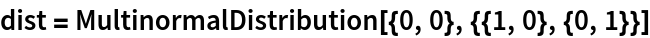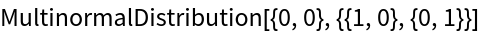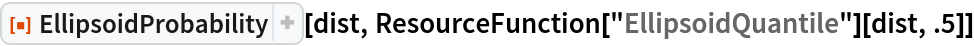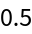Function Repository Resource:

# EllipsoidProbability

Find the cumulative probability within the specified elliptic domain

Contributed by: Wolfram Research
 ResourceFunction["EllipsoidProbability"][dist,ellipse] gives the cumulative probability of dist over ellipse centered at the mean of dist.

## Details and Options

ResourceFunction["EllipsoidProbability"] is defined for elliptically contoured multivariate distributions such as MultinormalDistribution or MultivariateTDistribution.
Typically, ellipse is an Ellipsoid object obtained via EllipsoidQuantile.

## Examples

### Basic Examples (1)

The probability inside an ellipsoid for a bivariate normal distribution:

 In:=Out=In:=Out=## Requirements

Wolfram Language 11.3 (March 2018) or above

## Version History

• 1.0.0 – 19 February 2019